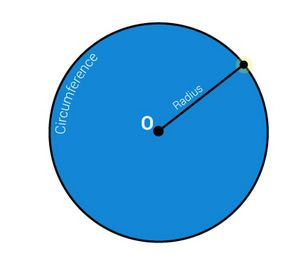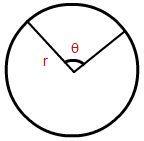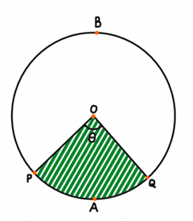# Area Of A Sector Of A Circle Formula

### Circle-

A circle is a geometrical shape which is made up of an infinite number of points in a plane that are located at a fixed distance from a point called as the centre of the circle. The fixed distance from any of these points to the centre is known as the radius of the circle.### Arc-

A  part of a curve lying on the circumference of a circle.Length of an arc of a sector- The length of an arc is given as-

$$\begin{array}{l}\frac{\theta }{360} \times 2 \pi r\end{array}$$

There are instances where the angle of a sector might not be given to you. Instead, the length of the arc is known. In such cases, you can compute the area by making use of the following.

### Sector-

A sector is a portion of a circle which is enclosed between its two radii and the arc adjoining them. The most common sector of a circle is a semi-circle which represents half of a circle.

A circle containing a sector can be further divided into two regions known as a Major Sector and a Minor Sector.

In the figure below, OPBQ is known as the Major Sector and OPAQ is known as the Minor Sector. As Major represent big or large and Minor represent Small, which is why they are known as Major and Minor Sector respectively. In a semi-circle, there is no major or minor sector.We know that a full circle is 360 degrees in measurement. Area of a circle is given as π times the square of its radius length. So if a sector of any circle of radius r measures θ, area of the sector can be given by:

Area of sector =

$$\begin{array}{l}\frac{\theta }{360} \times \pi r^{2}\end{array}$$

Derivation:

In a circle with centre O and radius r, let OPAQ be a sector and θ (in degrees) be the angle of the sector.

Area of the circular region is πr².

Let this region be a sector forming an angle of 360° at the centre O.
Then, the area of a sector of circle formula is calculated using the unitary method.
When the angle at the centre is 360°, area of the sector, i.e., the complete circle = πr²
When the angle at the center is 1°, area of the sector =

$$\begin{array}{l}\frac{\pi .r ^{2}}{360^{0}}\end{array}$$

Thus, when the angle is θ, area of sector, OPAQ =
$$\begin{array}{l}\frac{\theta }{360^{o}}\times \pi r^{2}\end{array}$$

Solved Examples:

Questions 1: For a given circle of radius 4 units, the angle of its sector is 45°. Find the area of the sector.

Solution: Given, radius r = 4 units

Angle θ = 45°

Area of the sector =

$$\begin{array}{l}\frac{\theta }{360^{o}}\times \pi r^{2}\end{array}$$

=

$$\begin{array}{l}\frac{45^{0}}{360^{0}}\times\frac{22}{7}\times 4^{2}=6.28\;sq.units\end{array}$$

Questions 2: Find the area of the sector with a central angle of 30° and a radius of 9 cm.

Solution: Given,

Angle θ = 30°

Area of the sector =

$$\begin{array}{l}\frac{\theta }{360^{0}}\times \pi r^{2}\end{array}$$

=

$$\begin{array}{l}\frac{30^{0}}{360^{0}}\times \frac{22}{7}\times 9^{2}=21.21cm^{2}\end{array}$$

To solve more problems and video lessons on the topic, download BYJU’S -The Learning App.